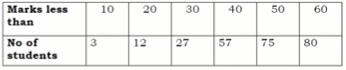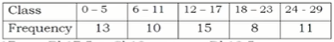Saturday, December 4, 2021
Home > CBSE Class 10 > Statistics Mean Median Mode Class 10 Maths MCQ Questions with Answer Keys

# Statistics Mean Median Mode Class 10 Maths MCQ Questions with Answer KeysHi students, Welcome to AMBiPi (Amans Maths Blogs). In this article, you will get Statistics Mean Median Mode Class 10 Maths MCQ Questions with Answer Keys. You can download this PDF and save it in your mobile device or laptop etc.

Statistics MCQ Questions for Class 10 Question No 11:

In the figure of more than and less than ogive curve as below, the value of the median isOption A : 5

Option B : 40

Option C : 80

Option D : 15

Option D : 15

Statistics MCQ Questions for Class 10 Question No 12:

In the following data, the modal class of the data isOption A : 10 – 20

Option B : 20 – 30

Option C : 30 – 40

Option D : 50 – 60

Option C : 30 – 40

Statistics MCQ Questions for Class 10 Question No 13:

If mode of a data is 45 and its mean is 27, then the median of the data is

Option A : 30

Option B : 27

Option C : 33

Option D : None of these

Option C : 33

Statistics MCQ Questions for Class 10 Question No 14:

For a symmetrical distribution of data, which of the following is correct.

Option A : Mean > Median > Mode

Option B : Mean < Mode < Median

Option C : Mode = (Mean + Median) / 2

Option D : Mean = Median = Mode

Option D : Mean = Median = Mode

Statistics MCQ Questions for Class 10 Question No 15:

If the mode of the following data is 7, then find the value of k.

2, 4, 6, 7, 5, 6, 10, 6, 7, (2k + 1), 9, 7, 13

Option A : 3

Option B : 7

Option C : 4

Option D : 2

Option A : 3

Statistics MCQ Questions for Class 10 Question No 16:

The upper limit of the median class of the following distribution of the data.Option A : 17

Option B : 17.5

Option C : 18

Option D : 18.5

Option B : 17.5

Statistics MCQ Questions for Class 10 Question No 17:

The measure of central tendency is given by x-coordinate of the point of intersection of the more than and less than ogive curve is

Option A : Mean

Option B : Median

Option C : Mode

Option D : All of Above

Option B : Median

Statistics MCQ Questions for Class 10 Question No 18:

A data has 25 observations (arranged in ascending order). Which observation represents the median

Option A : 12th observation

Option B : 13th observation

Option C : 14th observation

Option D : 15th observation

Option B : 13th observation

Statistics MCQ Questions for Class 10 Question No 19:

For a give data with 40 observation, the less than ogive and the more than ogive curve interest at (20.5, 1). The median of the data is

Option A : 5.5

Option B : 10.5

Option C : 15.5

Option D : 20.5

Option D : 20.5

Statistics MCQ Questions for Class 10 Question No 20:

The algebraic sum of the deviation of a frequency distribution from its mean is

Option A : Always positive

Option B : Always negative

Option C : 0

Option D : None of these

Option C : 0

CBSE Class 10 Maths Chapterwise MCQs
1 : Real Numbers MCQ
| 1 to 10 | 11 to 20 | 21 to 30 | 31 to 40 | 41 to 50
2 : Polynomials MCQ
| 1 to 10 | 11 to 20 | 21 to 30 | 31 to 40 | 41 to 50
3 : Linear Equations MCQ
| 1 to 10 | 11 to 20 | 21 to 30 | 31 to 40 | 41 to 50
| 1 to 10 | 11 to 20 | 21 to 30 | 31 to 40 | 41 to 50
5 : Arithmetic Progression MCQ
| 1 to 10 | 11 to 20 | 21 to 30 | 31 to 40 | 41 to 50
6 : Triangles MCQ
| 1 to 10 | 11 to 20 | 21 to 30 | 31 to 40 | 41 to 50
7 : Coordinate Geometry MCQ
| 1 to 10 | 11 to 20 | 21 to 30 | 31 to 40 | 41 to 50
8 : Introduction to Trigonometry MCQ
| 1 to 10 | 11 to 20 | 21 to 30 | 31 to 40 | 41 to 50
9 : Application of Trigonometry MCQ
| 1 to 10 | 11 to 20 | 21 to 30 | 31 to 40 | 41 to 50
10 : Circles MCQ
| 1 to 10 | 11 to 20 | 21 to 30 | 31 to 40 | 41 to 50
11 : Constructions MCQ
| 1 to 10 | 11 to 20
12 : Area Related to Circles MCQ
| 1 to 10 | 11 to 20 | 21 to 30 | 31 to 40 | 41 to 50
13 : Surface Area & Volume MCQ
| 1 to 10 | 11 to 20 | 21 to 30 | 31 to 40 | 41 to 50
14 : Statistics MCQ
| 1 to 10 | 11 to 20 | 21 to 30 | 31 to 40 | 41 to 50
15 : Probability MCQ
| 1 to 10 | 11 to 20 | 21 to 30 | 31 to 40 | 41 to 50
16 : Previous Year Maths MCQs from CBSE Board Class 10
| 1 to 100
AMBiPi
error: Content is protected !!